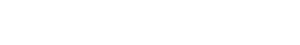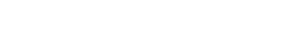# Earnings, Simple and Compound Interest | Cambridge IGCSE Mathematics[Please watch the video attached at the end of this blog for a visual explanation of earnings, simple, and compound interest]

In today’s world, this lesson is extremely important. You might not be asked about the area of a triangle in your day-to-day life once you become an adult, but learning about earnings, simple interest, and compound interest is crucial as this is something you will get to use in your everyday life.

So to begin with, we are going to look at profit and interest, so basically profit and loss percentages.

## Profit and interest

To begin things, we will first take a look at profit and interest, or profit and loss percentages. We learned in the previous lesson Fractions, Decimals, and Percentages, that a percentage can also be expressed as a fraction of a hundred (i.e. 60% is also equal to 60⁄100) as “percent” means one part in every hundred.

Example problem:

A television set was bought for \$3900, \$200 was spent on transportation, and \$900 on the repair. It was sold at a loss of 10%. Find the selling price of the television.

In this problem question, the first thing you must do is find out the total cost.

Total cost = Actual buying price + Repair + Transport

This means the total cost of the television is \$5000. From this amount, it says that they have lost 10% so we can calculate the 10% loss as

Loss = 10⁄100 × 5000 = \$500.

Therefore, the selling price of the television will therefore be

Total cost – Loss = \$5000 – \$500 = \$4500.

## Reverse Percentage

Initially, we were trying to find the price after a particular discount, sale, or loss. Here, we are trying to work backward, therefore these are known as reverse percentages. Therefore if there is a said amount as the sale price, and there is a said amount as the gained price the question that would be asked is “how much was the original?”

Example problem:

A bed sheet was sold for \$483 thereby gaining 15%. Find the cost price of the bed sheet.

The amount of \$483 is including the 15% profit. The question asks us to find the cost price of the bedsheet, that is, what the original price of the bedsheet was.

The total percentage we have here is 115%. Therefore, unlike earlier where we were multiplying by the percentage/100, here you must multiply by 100/percentage.

This gives us  100⁄115, and once this equation is simplified, you would end up with \$420.

## Simple interest

This is something very common when talking about money, and there is a very simple formula that we can use in finding this out.The principal is the amount that you initially invest and the rate is simply the interest rate mentioned in the problem.

If you look at the example given below, you can see that once you substitute the given values in the required places, the answer can be obtained quite easily.Keep in mind however that this amount (\$930 in the example) is only the interest, therefore if you were asked to find out the total amount of money they have at the end of this period, then you need to add the amount earned through the interest (\$930) to the initial principal amount (\$6200)

## Compound interest

Compound interest is when you earn money on both the interest you have saved and the interest that you earned.

This is a bit more complicated than simple interest, but once you get the hang of it you can do it pretty quickly!

Compound interest also relies on a formula.If we look at the example below, and you substitute the given values (principal = \$30,000, time = 6 years, rate = 1.8), then too, you can get the answer pretty soon.We therefore simply substitute in the rate as 1.8, the time as a power value (6), and the principal as 30,000.

Here, be mindful of breaking it down in an order that the calculator would be able to solve.

## Helpful points for practicing earnings, simple, and compound interest for your upcoming Cambridge IGCSE Mathematics exams.

Just like any other subject, Mathematics relies heavily on how well you prepare in advance for your exams. To make things easier, here are a few pointers that may help you get ready for your upcoming Cambridge IGCSE Mathematics exams.

Study the difference between simple interest and compound interest thoroughly.

Practice sample questions – Practise, practice, practice. Simple interest questions and compound interest questions might appear simple, but can sometimes be very tricky. Our question banks have questions of different difficulty levels that you can practice before your actual exams.

Practice past paper questions to familiarise yourself with the format of the questions and paper.

Ask for help – You are never too late to ask for assistance if you’re struggling. Reach out to us at Tutopiya to find the right tutor to help you pass your exams with flying colors!

Watch the video below for a visual explanation of earnings, simple interest, compound interest, and a few calculator tips as well. Then attempt the quiz to challenge yourself!